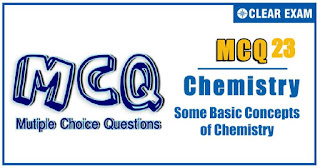## [LATEST]\$type=sticky\$show=home\$rm=0\$va=0\$count=4\$va=0

IIT JEE exam which consists of JEE Main and JEE Advanced is one of the most important entrance exams for engineering aspirants. The exam is held for candidates who are aspiring to pursue a career in the field of engineering and technical studies. Chemistry is important because everything you do is chemistry! Even your body is made of chemicals. Chemical reactions occur when you breathe, eat, or just sit there reading. All matter is made of chemicals, so the importance of chemistry is that it's the study of everything..

Q1.[Na^+ ] in a solution prepared by mixing 30.00 mLof 0.12 M NaClwith 70 mLof 0.15 M Na_2 SO_4 is :
•  0.135 M
•  0.141 M
•  0.210 M
•  0.246 M
Solution
(d) NaCl⇌Na^++Cl^- Na_2 SO_4⇌2Na^++SO_4^(2-) [Na^+ ]_( mix)=(30×0.12+70×0.15×2)/(30+70)=0.246 M

Q2.A metal nitrate reacts with KI to give a black precipitate which on addition of excess of KI converts into orange colour solution. The cation of metal nitrate is
•  Hg^(2+)
•  Bi^(3+)
•  Pb^(2+)
•  Pb^(2+)
Solution
(b) Bi^(3+)+3I^-⟶Bil_3↓ black ppt Bil_3+KI⟶K[Bil]_4 orange solution

Q3.Upon treatment with ammoniacal H_2 S, the metal ion that precipitates as a sulphide is
•  Fe (III)
•  Al (III)
•  Mg (II)
•  Zn (II)
Solution
option d

Q4.BIf two compounds have same empirical formula but different molecular formula, they must have
•  Same viscosity
•  Same vapour density (VD)
•  Different molecular weight
•  Different percentage composition
Solution
(c) Same empirical formula, it means ratio of atoms is identical. Hence, they differ in molecular weight

Q5.8A gaseous mixture contains oxygen and nitrogen in the ratio 1:4 by weight. Therefore, the ratio of the number of molecules is:
•  1:4
•  1:8
•  7:32
•  3:16
Solution
(c) Mol. ratio O_2:N_2 1/32:4/28 Molecules N_A/32 ∶(4N_A)/28⇒7∶32

Q6.Analysis of chlorophyll shows that it contains 2.40 per cent magnesium. Thus, number of atoms in 1 g chlorophyll is
•  0.001
•  1.00
• 6.02×10^20
•  1.445×10^19
Solution
(c) Mg in 1 g chlorophyll= (1×2.4)/100 g =(1×2.4)/(100×24) mol =(1×2.4×6.02×10^23)/(100×24) atoms =6.02×10^20 atoms

Q7.To make 0.01 mole which of the following has maximum mass?
•  NaHCO_3
•  Na_2 CO_3
•  Na_2 SO_4
•  Na_2 C_2 O_4
Solution
(c) Molar mass 0.01 mol (a) NaHCO_3=84 g 0.84 g (b) Na_2 CO_3=106 g 1.06 g (c) Na_2 SO_4=142 g 1.42 g (d) Na_2 C_2 O_4=134 g 1.34 g

Q8.Select the correct statement
•  In iodometric titration, hypo is taken in burette
•  In iodimetric titration, ---- I_2solution is taken in burette
•  In iodometric titration ----- I_2formed exist as I_3^-
•  All the above are correct statements
Solution
(d) (a) CuSO_4+4Kl→Cu_2 l_2+2K_2 SO_4+l_2 l_2+2Na_2 S_2 O_3→2Nal+Na_2 S_4 O_6 Liberated l_2 is estimated by Na_2 S_2 O_3(hypo) taken in burette Thus, true (b) l_2 (in burette) +2N_2 S_2 O_3→2Nal+Na_2 S_4 O_6 Thus, true (c) l_2+Kl⇌Kl_3 Thus, true

Q9. Precipitates of IIA and IIB can be separated by
•  NaOH
•  Yellow (NH_4 )_2 S
•  Both (a) and (b)
•  None of these
Solution
option c

Q10. What is the valency of an element of which the equivalent weight is 12 and the specific heat is 0.25?
•  1
•  2
•  3
• 4
Solution
(b) Specific heat × Atomic weight = 6.4 (Dulong and Petit law) Atomic weight = 6.4/(specific heat)=6.4/0.25 Atomic weight = Ew× valency = 25.6 Valency =(Atomic weight)/Ew=25.6/12=2 (Valency is always a whole number)#### Written by: AUTHORNAME

AUTHORDESCRIPTION## Want to know more

Please fill in the details below:

## Latest NEET Articles\$type=three\$c=3\$author=hide\$comment=hide\$rm=hide\$date=hide\$snippet=hide

Name

ltr
item
BEST NEET COACHING CENTER | BEST IIT JEE COACHING INSTITUTE | BEST NEET & IIT JEE COACHING: SOME BASIC CONCEPTS OF CHEMISTRY Quiz-23
SOME BASIC CONCEPTS OF CHEMISTRY Quiz-23
https://1.bp.blogspot.com/-ceAZqyBVn-c/YPRvK5qZ2yI/AAAAAAAAYos/ehOxSd0mL900_QjmV6uxYVVXS_l8UmrTwCLcBGAsYHQ/s320/Quiz%2BImage%2B20%252822%2529.jpg
https://1.bp.blogspot.com/-ceAZqyBVn-c/YPRvK5qZ2yI/AAAAAAAAYos/ehOxSd0mL900_QjmV6uxYVVXS_l8UmrTwCLcBGAsYHQ/s72-c/Quiz%2BImage%2B20%252822%2529.jpg
BEST NEET COACHING CENTER | BEST IIT JEE COACHING INSTITUTE | BEST NEET & IIT JEE COACHING
https://www.cleariitmedical.com/2021/07/some-basic-concepts-of-chemistry-quiz-23.html
https://www.cleariitmedical.com/
https://www.cleariitmedical.com/
https://www.cleariitmedical.com/2021/07/some-basic-concepts-of-chemistry-quiz-23.html
true
7783647550433378923
UTF-8

STAY CONNECTED